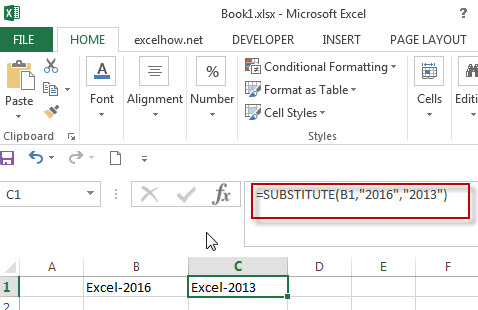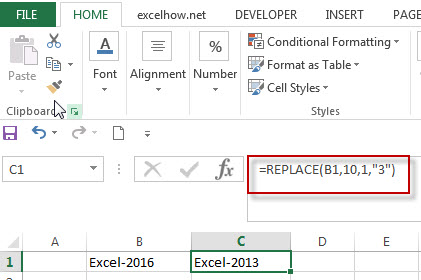# The difference between Replace function and Substitute function in Excel

There are two similar functions to replace text string in Excel. They are REPLACE and SUBSTITUTE functions. What’s the difference between REPLACE function and SUBSTITUTE function in excel 2013?  This post will introduce the difference between those two function in excel.

## SUBSTITUTE Function

The Excel SUBSTITUTE function replaces a new text string for an old text string in a text string.

The syntax of the SUBSTITUTE function is as below:

`= SUBSTITUTE(text, old_text, new_text,[instance_num])`

## REPLACE Function

The Excel REPLACE function replaces all or part of a text string with another text string

The syntax of the REPLACE function is as below:

`= REPLACE(old_text, start_num, num_chars, new_text)`

## The difference between Replace function and Substitute function

The replace function just replace text string in a specified position of a string. So when you want to replace text string, you must specified the position of the string that you want to replace.

The Substitute function will replace one or more specified text string in a given text string, so it will perform the lookup operation to search the text string that you want to replace. If yes, then replace it using the given new text string.

Let’s see the below examples:

1# if you want to replace text string and you already know the text to be replaced, then you need to use SUBSTITUTE function to replace the text.In the above example, the SUBSTITUTE function is going to search the old_text in the second argument, if it found the “2016”, then replace it with new_text “2013”.

2# if you know the position of the text that you want to replace, and you can use the REPLACE function.### Related Functions

• Excel Replace function
The Excel DATE function returns the serial number for a date.The syntax of the DATE function is as below:= DATE (year, month, day) …
• Excel Substitute function
The Excel SUBSTITUTE function replaces a new text string for an old text string in a text string. ….

Sidebar